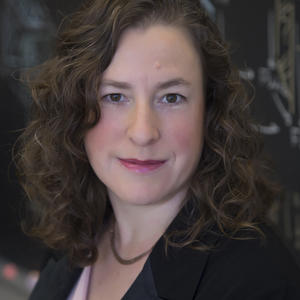## Kristine Bauer, Phd

PhD in Mathematics University of Illinois, Urbana, USA
B. A. in Mathematics, with honours Vassar College, USA### Areas of Research

Algebraic topology and homotopy theory
Algebraic topology is the study of topological spaces using algebraic invariants. Homotopy theory has its roots in the homotopy groups of spaces, and is one of the most important invariants in topology. The methods used to study the homotopy types of topological spaces were greatly generalized, especially after the pioneering work of Daniel Quillen. Now these homotopy theoretic tools are used in many other areas of mathematics, including algebra, algebraic geometry, physics, and category theory. I am especially interested in homotopy invariant functors, which are the generalization of algebraic invariants for any kind of homotopy category. Functor calculus refers to a method of approximating very complicated homotopy functors by simpler ones, and was pioneered by Tom Goodwillie. My research involves developing and using an algebraic version of Goodwillie's functor calculus.

### Supervising degrees

Math and Statistics - Doctoral: Unavailable
Math and Statistics - Masters: Seeking Students